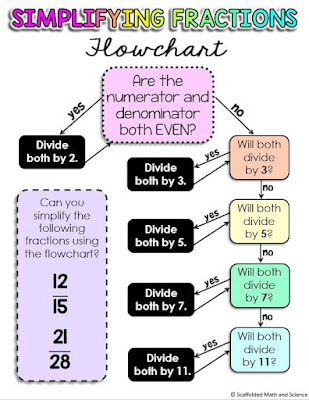## Pages

### Simplifying fractions using visual models and primes video

My favorite way to simplify fractions is by working through a list or prime numbers, checking if both numerator and denominator divide by each larger prime. I like this method because it makes simplifying fractions super concrete for students who need it. It also helps eliminate errors that can occur when a factor is missed. Students who aren't as confident with their division facts can even use a calculator (or divisibility rules) to check each prime.

After posting the left video above, I was asked on Instagram if I could explain why this method works. So I made the video on the right showing how to simplify fractions using visual models. Dividing both the numerator and denominator by 2, 3, 5, etc. is the same as dividing by 1 over and over again. Except in our case, those ones are in disguise as 2/2, 3/3, etc.

If you'd like to show this example in class, the dot sheet from the video is here in my dropbox.

To simplify the fraction 90/126 using primes, we first want to know if both the numerator and denominator are even. They are, so our fraction simplifies to 45/63. We know that both 45 and 63 are not even, so we move on to our next prime 3. "Do 45 and 63 both divide by 3?" They do, so our fraction then further simplifies to 15/21. Both 15 and 21 are still divisible by 3, so after dividing by 3 again we get 5/7.Simplifying fractions flowchart

This (free) simplifying fractions flowchart walks students through how to simplify fractions using prime numbers.

Browse all fraction activities